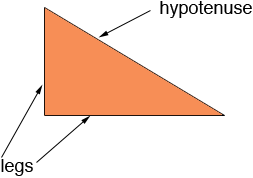SEARCH HOMEMath Central Quandaries & QueriesQuestion from Kasey: What is the hypotenuse of 96 squared and 108 squared?Hi Kasey,

The hypotenuse is the side of a right triangle that is opposite the right angle. The other two sides are sometimes called legs.The theorem of Pythagoras states that is the legs of a right triangle have lengths a and b and the hypotenuse has length c then a2 + b2 = c2, in other words a squared plus b squared equals c squared.

It looks to me that you have a right triangle with legs of length 96 and 108 units. If this is the case the hypotenuse has length c where

962 + 1082 = c2

thus

c2 = 9216 + 11664 = 20880

and hence

c = √20880 = 144.499 units

PennyMath Central is supported by the University of Regina and The Pacific Institute for the Mathematical Sciences.